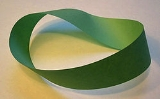xTopologyOverview

Topology is a major area of mathematics
Mathematics
Mathematics is the study of quantity, space, structure, and change. Mathematicians seek out patterns and formulate new conjectures. Mathematicians resolve the truth or falsity of conjectures by mathematical proofs, which are arguments sufficient to convince other mathematicians of their validity...

concerned with properties that are preserved under continuous
Continuous function
In mathematics, a continuous function is a function for which, intuitively, "small" changes in the input result in "small" changes in the output. Otherwise, a function is said to be "discontinuous". A continuous function with a continuous inverse function is called "bicontinuous".Continuity of...

deformations of objects, such as deformations that involve stretching, but no tearing or gluing. It emerged through the development of concepts from geometry
Geometry
Geometry arose as the field of knowledge dealing with spatial relationships. Geometry was one of the two fields of pre-modern mathematics, the other being the study of numbers ....

and set theory
Set theory
Set theory is the branch of mathematics that studies sets, which are collections of objects. Although any type of object can be collected into a set, set theory is applied most often to objects that are relevant to mathematics...

, such as space, dimension, and transformation.

Ideas that are now classified as topological were expressed as early as 1736. Toward the end of the 19th century, a distinct discipline developed, which was referred to in Latin as the geometria situs (“geometry of place”) or analysis situs (Greek-Latin for “picking apart of place”).